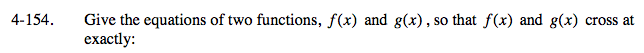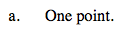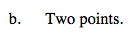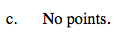### Home > A2C > Chapter 4 > Lesson 4.3.2 > Problem4-154

4-154.
1. Give the equations of two functions, f (x) and g(x), so that f (x) and g(x) cross at exactly: 4-154 HW eTool (Desmos). Homework Help ✎

1. One point.

2. Two points.

3. No points.There is more than one answer to each of the following situations. Only check one box at a time and be sure to make up your own equations.

Use the eTool below to view one example of each situation.
Click the link at right for the full version of the eTool: A2C 4-154 HW eTool